Next: Energy Density Up: Definitions of Number and Previous: Program Variables

### Number and Energy Spectra in One and Two Dimensions

The ordinary power spectraand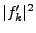are output in the same way regardless of the number of dimensions, but the spectra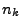andhave well defined normalizations, so in one and two dimensions they are normalized so as to try to approximate the values that they would have in three dimensions. Letbe the Fourier transform of a three dimensional field, and let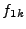andbe the Fourier transforms of the field taken on one and two dimensional slices respectively. Assuming that isotropy holds on average we show in section 6.3.5 that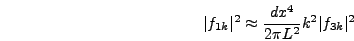(5.36)

and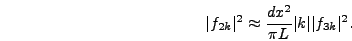(5.37)

Recall that the wave vectoris given by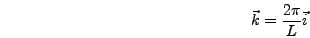(5.38)

whereis the position on the grid in Fourier space (i.e. a triplet of integers from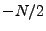to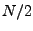). Thus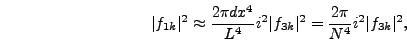(5.39)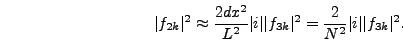(5.40)

The Fourier transformthat is actually calculated in one and two dimensional simulations will correspond toand. The definitions ofand, however, are given in terms of. Thus equations [5.33] and [5.34] are multiplied by prefactors equal to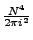and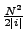in one and two dimensions respectively.Next: Energy Density Up: Definitions of Number and Previous: Program Variables